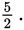# SAT Math Multiple Choice Question 319: Answer and Explanation

### Test Information

Question: 319

4.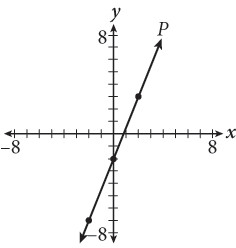Line P is shown in the coordinate plane here. If line Q (not shown) is the result of translating line P left 4 units and down 3 units, then what is the slope of line Q?

• A.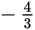• B. -1
• C.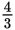• D.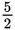Getting to the Answer: Find the slope of line P by counting the rise and the run from one point to the next, and you'll have your answer. From the y-intercept (0, -2), the line rises 5 units and runs 2 units to the point (2, 3), so the slope is